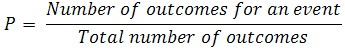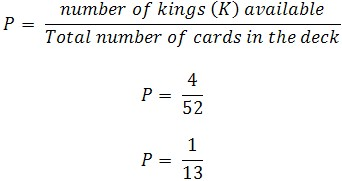Probability

Probability

The probability of an outcome for a given event can be numerically interpreted as a number between 0 and 1. If the outcome is more likely to occur, then the probability value will be closer to one.  If the outcome is less likely to occur, then the value will be closer to 0.

Theoretical probability (P) is calculated by:Example

One playing card is drawn from a standard deck of cards. What is the probability that the card drawn will be a king (K)?

There are 52 cards in a deck and there are four kings (K), one of each suit: